# Lambert series

(diff) ← Older revision | Latest revision (diff) | Newer revision → (diff)

The series of functions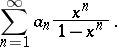(1)

It was considered by J.H. Lambert (see ) in connection with questions of convergence of power series. If the series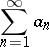converges, then the Lambert series converges for all values ofexcept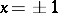; otherwise it converges for those values offor which the series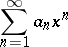converges. The Lambert series is used in certain problems of number theory. Thus, for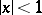the sumof the series (1) can be represented as a power series: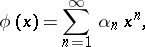(2)

whereand the summation is over all divisorsof. In particular, if, then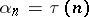, the number of divisors of; if, then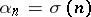, the sum of the divisors of. The behaviour of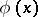(with suitable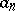) as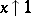is used, for example (see ), in the problem of Hardy and Ramanujan on obtaining an asymptotic formula for the number of "unbounded partitions" of a natural number.

How to Cite This Entry:
Lambert series. Encyclopedia of Mathematics. URL: http://encyclopediaofmath.org/index.php?title=Lambert_series&oldid=13504
This article was adapted from an original article by M.I. Voitsekhovskii (originator), which appeared in Encyclopedia of Mathematics - ISBN 1402006098. See original article